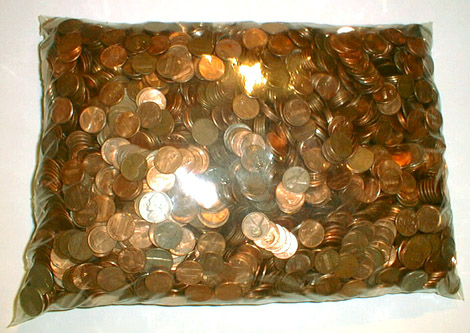to english | index | next

5020枚の硬貨から成る55米ドル64セント (金額第二九番)
US\$55.64 Which Consists of 5020 Coins (Money Amount No. 29)

The U. S. coins, a plastic bag
2002

cf. 5020 and 5564 are the fourth smallest amicable pair which Euler found in 1747.
(Two numbers are called amicable if each equals to the sum of the divisors of the other.)
Divisors of 5020: 1 + 2 + 4 + 5 + 10 + 20 + 251 + 502 + 1004 + 1255 + 2510 = 5564
Divisors of 5564: 1 + 2 + 4 + 13 + 26 + 52 + 107 + 214 + 428 + 1391 + 2782 = 5020